机器学习算法之：KNN

• KNN，全称K-Nearest Neighbor，中文名为K近邻
• 思想极度简单，最基础的分类算法，非常适合入门
• 应用数学知识极少，近乎为零
• 效果却很好
• 可以解释机器学习算法使用过程中的很多细节问题，更完整的刻画机器学习应用的流程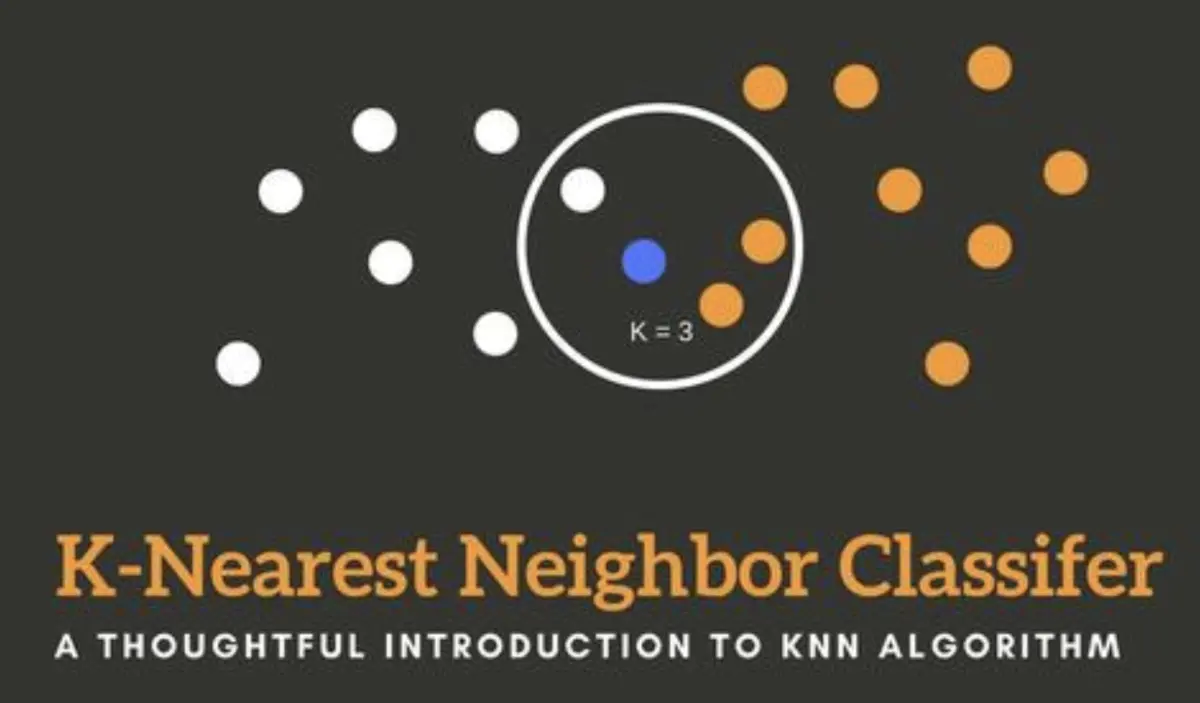KNN算法简介

• KNN，全称K-Nearest Neighbor，中文名为K近邻
• 思想极度简单，最基础的分类算法，非常适合入门
• 应用数学知识极少，近乎为零
• 效果却很好
• 可以解释机器学习算法使用过程中的很多细节问题，更完整的刻画机器学习应用的流程

KNN算法原理

下面我们开始用一个简单的例子来解释KNN的原理：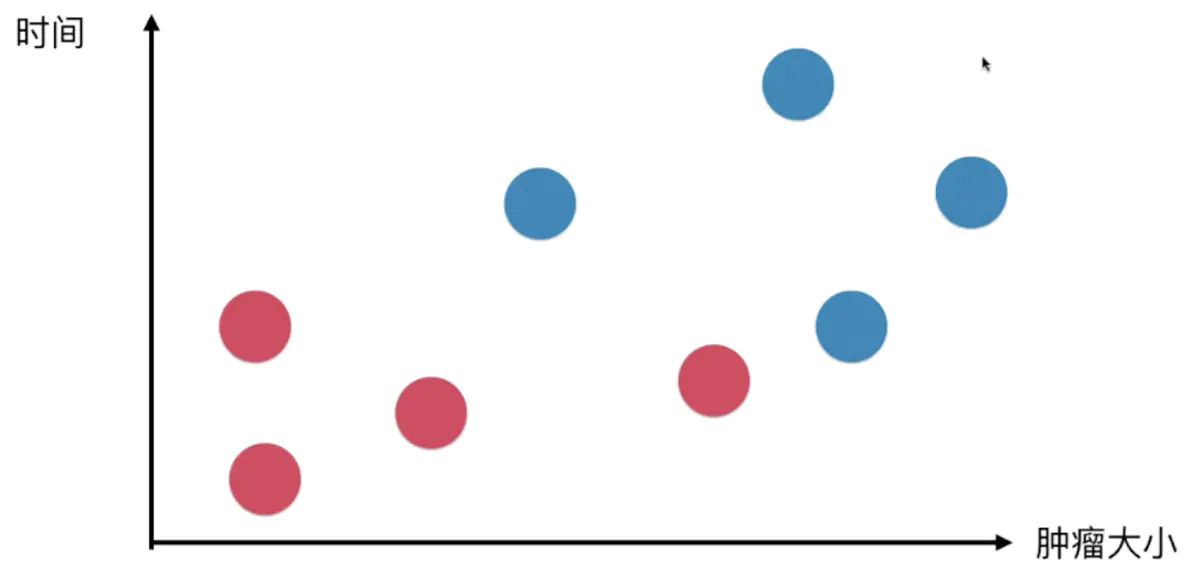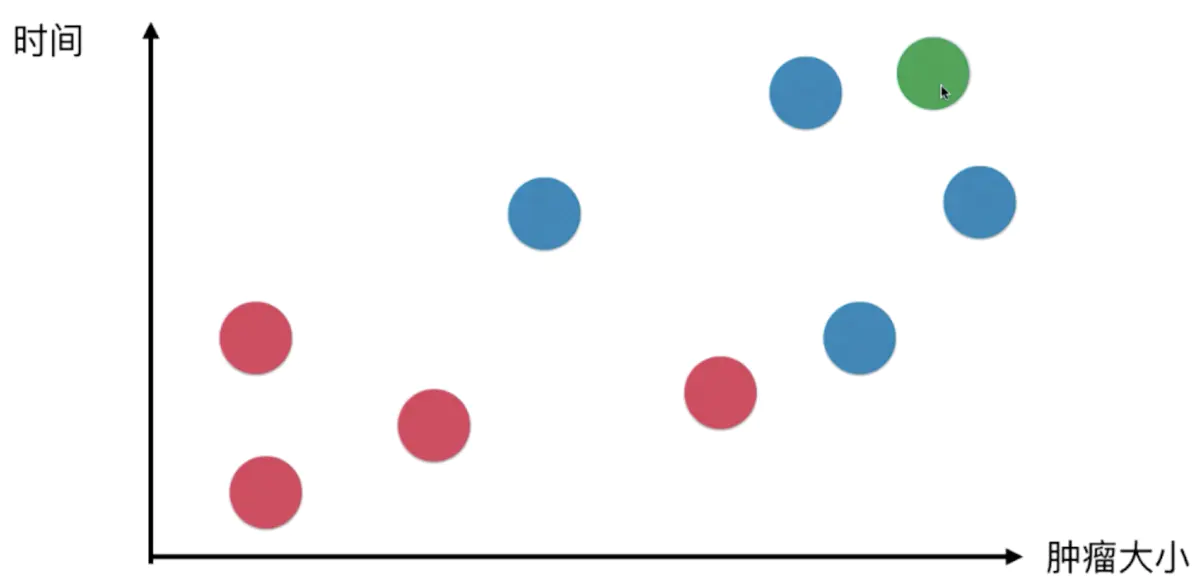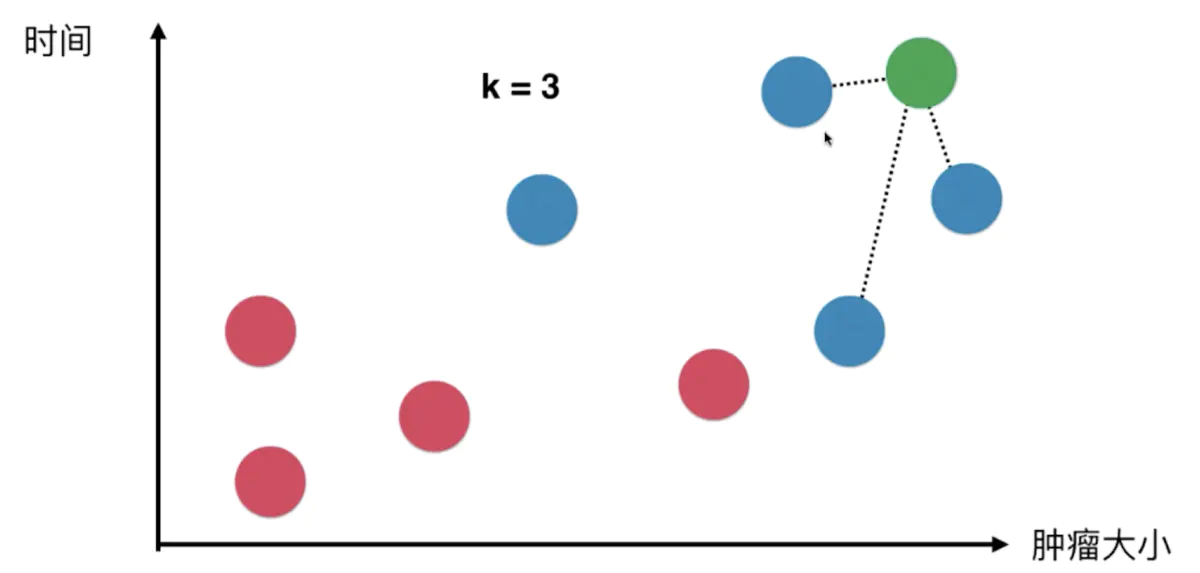关于k值与样本数据的距离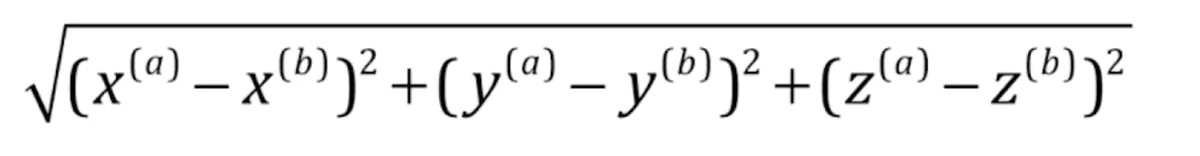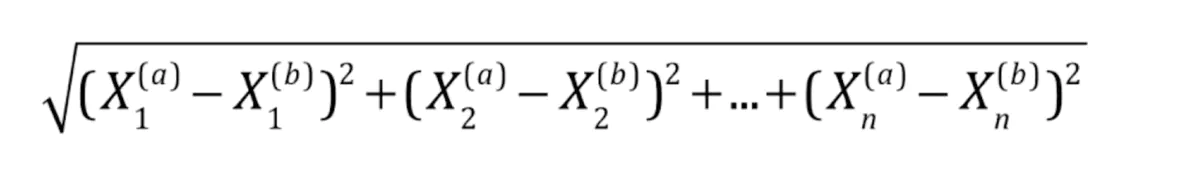算法实现

import numpy as np
import matplotlib.pyplot as plt

# 定义一组肿瘤的样本的特征
raw_data_X = [[3.39, 2.33],
[3.11, 1.78],
[1.34, 3.36],
[3.58, 4.67],
[2.28, 2.86],
[7.42, 4.69],
[5.75, 3.53],
[9.17, 2.51],
[7.79, 3.42],
[7.93, 0.79]]

# 对应样本已知的类别，0为良性，1为恶性
raw_data_y = [0, 0, 0, 0, 0, 1, 1, 1, 1, 1]

# 用numpy封装为训练集
X_train = np.array(raw_data_X)
y_train = np.array(raw_data_y)

# 绘制已知肿瘤分类为0和1的散点图
plt.scatter(X_train[y_train == 0, 0],
X_train[y_train == 0, 1], color='g')  # 绿色为良性
plt.scatter(X_train[y_train == 1, 0],
X_train[y_train == 1, 1], color='r')  # 红色为恶性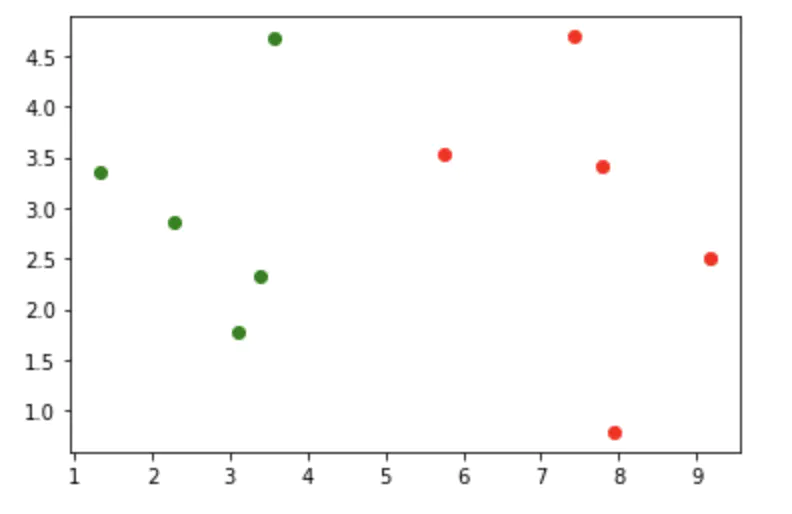# 现在新来的肿瘤
x_new = np.array([8.09, 3.34])

plt.scatter(X_train[y_train == 0, 0],
X_train[y_train == 0, 1], color='g')  # 绿色为良性
plt.scatter(X_train[y_train == 1, 0],
X_train[y_train == 1, 1], color='r')  # 红色为恶性

# 单独绘制一下新肿瘤
plt.scatter(x_new, x_new, color='b')  # 蓝色为新肿瘤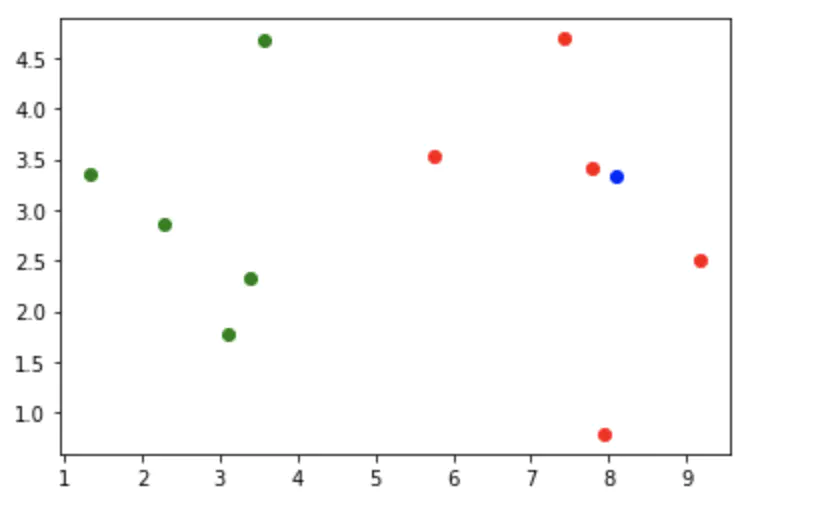# 手写一遍kNN算法过程
from collections import Counter
from math import sqrt

# 定义一个空的数组，用于保存新数据与已知样本数据的欧氏距离
distances = []

# 这里可以回顾一下欧氏距离的数学公式
for x_train in X_train:
d = sqrt(np.sum((x_train - x_new)**2))
distances.append(d)

# 此时的distances中就已经包含了新肿瘤与每一个样本的距离，打印一下看看
distances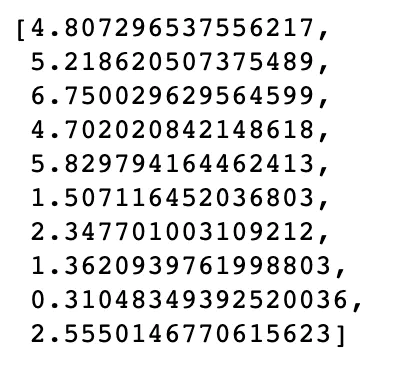# 可以用更简介生成表达式的语法计算
distances = [sqrt(np.sum((x_train-x_new)**2)) for x_train in X_train]

# 再打印一下看看，一样的吧
distances# 对距离排序看看
np.sort(distances)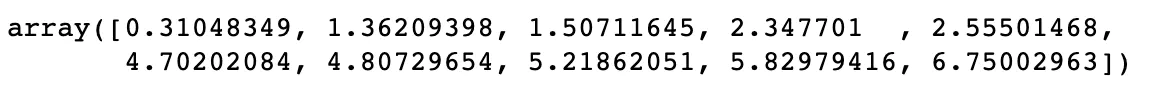# 但是现在我们需要的是：distance数组在排序后所对应排序前的index
np.argsort(distances)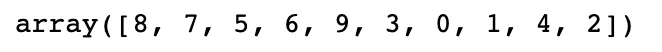# 我们将这个索引值保存为nearest变量
nearest = np.argsort(distances)

# 现在拍脑袋定义一个k值6，which means我们将比对距离新数据最近的6个样本，让这6个样本vote
k = 6

# 遍历nearest对象，将正确排序的索引引用到已知分类的结果集y，得到距离最近的6个y的结果
topk_y = [y_train[i] for i in nearest[:k]]

# 打印一下6个样本的投票结果，可以看到是分类1 VS 分类0是5：1
topk_y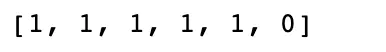# 调用一个计数函数，返回一个kv对象，会自动根据value将key从大到小排序，打印一下可以看到分类1有5票，分类0有1票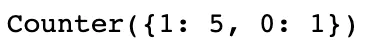# 由于刚才的结果是个对象，我们用most_common函数转换成tuple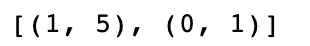# 由于我们只关心计数最多的那个key值（即分类结果），所以我们只需要取第一个tuple的key值

# 打印一下，新数据属于分类1，也就是恶性肿瘤
predict_y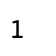scikit-learn中的KNN算法

from sklearn.neighbors import KNeighborsClassifier

KNN_classifier = KNeighborsClassifier(n_neighbors=6)
KNN_classifier.fit(X_train, y_train)

# 直接predict会报一个错，原因是x_new是一个一维向量，而X_train是一个二维矩阵，我们需要将x_new也reshape成一个一行的二维矩阵
y_predict = KNN_classifier.predict(x_new)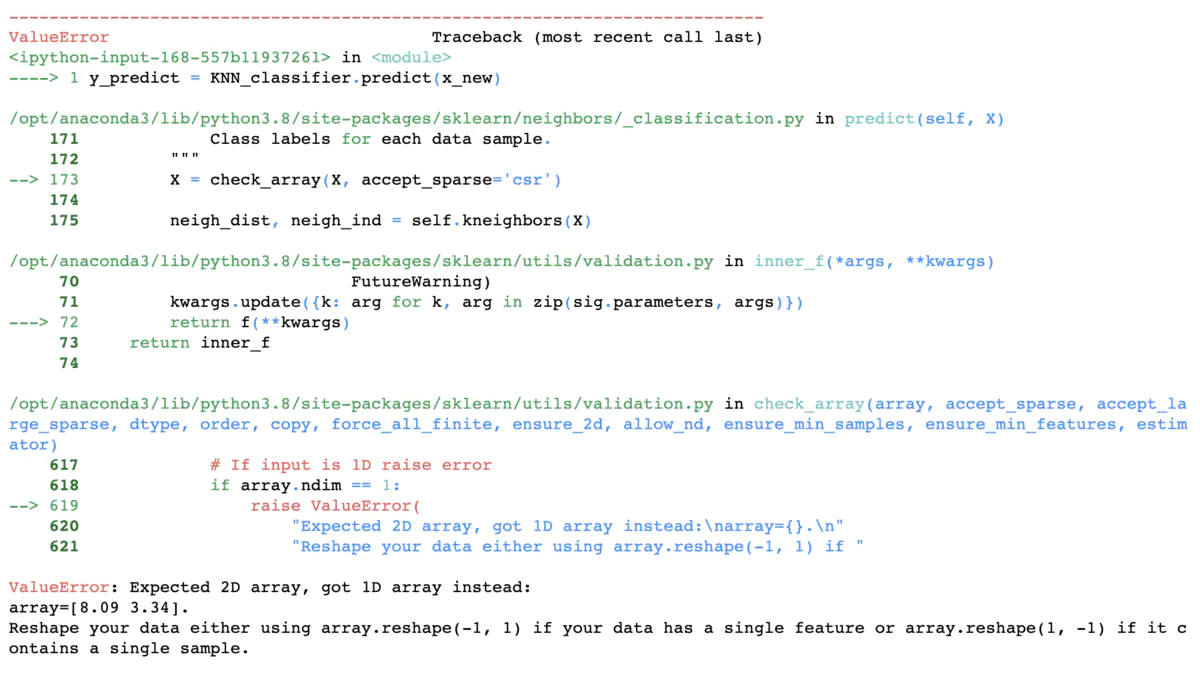y_predict = KNN_classifier.predict(x_new.reshape(1, -1))

# 再打印一下，problem solved
y_predict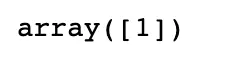# 强迫症同学还是取一下具体的值吧，就是1了
y_predict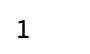/** 上述的predict会直接给出具体的分类值，但是我们也可以调用predict_proba看一下是0还是1的概率，可以看到0的概率是0.1666，1的概率是0.8333，也就是1：5啦**/
KNN_classifier.predict_proba(x_new.reshape(1, -1))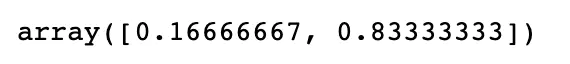小结

• KNN似乎不用建模？
• KNN算法是非常特殊的，可以认为是没有模型的算法
• 但是为了和其他算法统一，可以换个角度理解，认为训练的样本集就是模型本身

TBC……